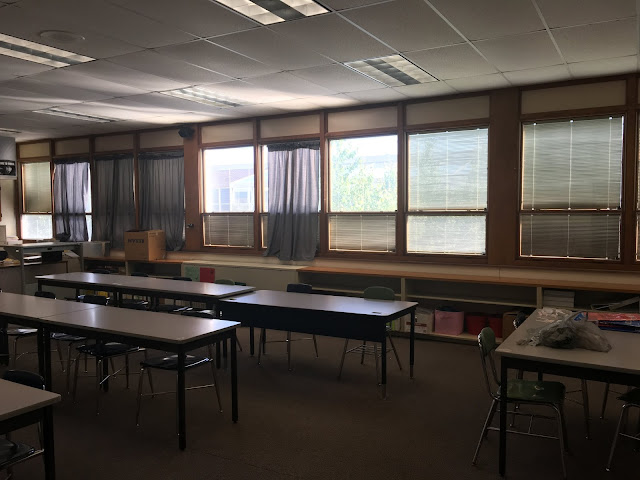Tuesday, November 25, 2014

Hidden Squares Activity - Equations with Variables on Both Sides

I did this activity last year with the snowflake posters if you want to check that out here.

To start class:

Tell the students there are a total of 32 small blue squares on this poster.  Some are hidden under the purple flaps.  Let them know that there are the same amount of square hidden under each flap.  How many blue squares are hidden under the flaps?

Most of my students noticed that there were 17 exposed blue squares and that meant that 15 were hidden.  If there are 5 flaps that means there must be 3 under each flap.

Me:  When we don't know the quantity or value of something what do we do?
Student:  We use x.
Me:  Right a variable.  Can we represent this poster as an expression using constants (numbers) and a variable?

The students were able to come up with 5x + 17 = 32, and then noticed that they needed to take exactly the same steps they took when they solved the poster problem above.

Me:  Do you see the "bubbles"?  What do you notice about the bubbles?
Student:  They have the same things inside them.
Me:  Let's use this information to write an equation.

With a little prodding the students came up with 2(2x+5) + x + 7 = 32
As we wrote each line of the solution, we made the connection with the poster to see where the numbers were coming from.

Taking Things Up a Notch:

Show the students this poster (without the algebra at first) and ask if they can determine how many squares are hidden under each flap.
They need to know that both posters have the same amount of small blue squares on them and the same amount are hidden under each purple flap.

All of my students used trial and error for this at first.  But then as a class we were able to solve with algebra.  I like that after we finish the algebra, we are able to lift a flap to see if we're right.

Now It's the Students' Turn:

I put students into groups of 2 to 3 and had them create their own posters.  The requirements were:
1) There must be the same number of small squares on each poster.
2) There must be the same number of small squares hidden under each flap.
3) Each poster must have a different amount of flaps.
I also wanted them to have "bubbles" on at least one of their posters.

The last image is very interesting.  The students and I had a nice discussion about it.  At first they said the answer was 1.  I agreed.  But then I asked if it could be 2.  They agreed.  3? 4? 5?  Why?  How can we write what all the answers are?  What does this look like algebraically?

Next time:

I enjoy this activity.  It's such a concrete way to talk about equations, the distributive property, combining like terms, and even consistent and inconsistent systems.  Next time I will focus more on the "why" on the poster requirements.

1) The posters must have the same amount of small squares.  Why?  What would happen if they didn't?

2) There must be the same number of squares under each flap.  Why?  What would happen if they didn't?

3) There must be different amount of flaps on each poster?  Why?  What would happen if they did?

1 comment:

1.I'm really loving this! Great job. So many possibilities for discussions and extensions. The physical-ness of this will be a seller for my grade 9 applieds. Thanks

Classroom Window Makeover

This is pretty much what my classroom has looked like for the past, oh 6 to 7 years.  I have this beautiful wall of windows and we receive ...